# How to calculate average (mean) in Excel - AVERAGE, AVERAGEIF, AVERAGEIFS formula examples

The tutorial shows how to calculate mean in Excel for different data types by using AVERAGE or AVERAGEA formulas. You will also learn how to use the AVERAGEIF and AVERAGEIFS functions to average cells that meet certain criteria.

In plain English, calculating the average for a set of values if finding out the most common value in the set. For example, if a few athletes have run a 100m sprint, you may want to know the average result - i.e. how much time most sprinters are expected to take to complete the race.

In mathematics, average is called the arithmetic mean, or simply the mean, and it is calculated by adding a group of numbers together and then dividing by the count of those numbers.

In the above example, if the first athlete covered the distance in 10.5 seconds, the second needed 10.7 seconds, and the third took 11.2 seconds, the average time would be 10.8 seconds:
`=(10.5+10.7+11.2)/3`.

To calculate average in Excel, you won't need to write such mathematical expressions, powerful Excel Average functions will do the work behind the scene. Further on in this tutorial, we will discuss the syntax of each function and illustrate it with examples of uses.

## Excel AVERAGE function

You use the AVERAGE function in Excel to return the average (arithmetic mean) of the specified cells.

AVERAGE(number1, [number2], …)

Number1, number2, … are numeric values for which you want to find the average. The first argument is required, subsequent ones are optional, and up to 255 arguments can be included in a single formula. The parameters can be supplied as numbers, cell references, or ranges.

### Using the AVERAGE function in Excel - formula examples

AVERAGE is one of the most straightforward and easy-to-use Excel functions, and the following examples prove this.

#### Example 1. Calculating an average of numbers

To find out an average of certain numbers, you can supply them directly in your Excel average formula. For example, `=AVERAGE(1,2,3,4)` returns 2.5 as the result.

To calculate a column average, supply a reference to the entire column:
`=AVERAGE(A:A)`

To get a row average, enter the row reference:
`=AVERAGE(1:A)`

To compute an average of numbers in a given range, specify that range in your Average formula:
`=AVERAGE(A1:C20)`

To return an average of non-adjacent cells, you supply each cell individually, e.g.
`=AVERAGE(A1, C1, D1)`

And naturally, nothing prevents you from including values, cells references and ranges in the same formula, as your business logic requires. For example, the following average formula calculates the average of 2 ranges and 1 individual cell:

`=AVERAGE(B3:B5, C7:D9, B11)`

Tip. If you want to round the returned number to the nearest integer, use one of the Excel rounding functions, for example: `=ROUND(AVERAGE(B3:B5, B7:B9, B11),0)`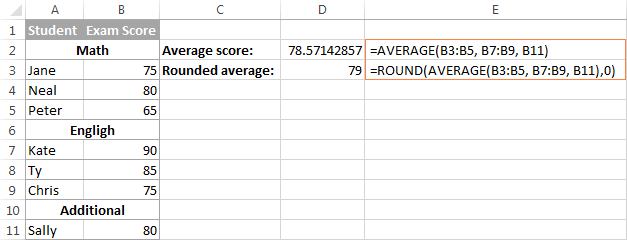Apart from numbers, you can use the Excel AVERAGE function to calculate an average of other numeric values such as percentages and times, as demonstrated in the following examples.

#### Example 2. Calculating average percentage in Excel

If you have a column of percentages in your sheet, how do you get an average percent rate? By using a normal Excel formula for average :)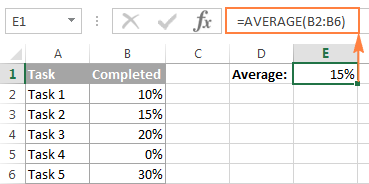Note. Please pay attention that the Excel AVERAGE function includes zero values when calculating an average. If you'd rather exclude zeros, use AVERAGEIF instead, as demonstrated in the following example.

#### Example 3. Calculating average time in Excel

As you may remember, at the beginning of this tutorial, we found out the average time of three 100m sprinters with a pretty simple calculation. But what if you want to average times that include hours, minutes and seconds? Calculating different time units manually, would be a real pain… but the AVERAGE formula in Excel copes perfectly.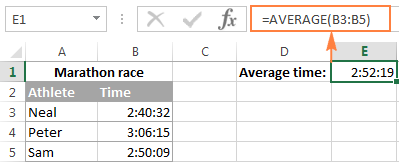### Excel AVERAGE function - things to remember!

As you've just seen, using the AVERAGE function in Excel is easy. However, it does have a few specificities that you need to be aware of.

• Cells with zero values (0) are included in the average.
• Cells containing text strings, Boolean values of TRUE and FALSE, and empty cells are ignored. If you want to include Boolean values and text representations of numbers in the calculation, use the AVERAGEA function.
• Boolean values that you type directly in the Excel AVERAGE formula are counted. For example, the formula `=AVERAGE(TRUE, FALSE)` returns 0.5, which is the average of 1 and 0.
Note. When using the AVERAGE function in Excel sheets, please do keep in mind the difference between cells containing zero values and blank cells - 0's are counted, but empty cells are not. This might be especially confusing if the "Show a zero in cells that have a zero value" option is unchecked in a given sheet. You can find this option under Excel Options > Advanced > Display options for this worksheet.

## AVERAGEA function in Excel

The AVERAGEA function is similar to Excel AVERAGE in that it calculates the average (arithmetic mean) of the values in its arguments. The difference is that AVERAGEA includes all non-empty cells in a calculation, whether they contains numbers, text, Boolean values of TRUE and FALSE, and empty strings returned by other formulas.

AVERAGEA(value1, [value2], …)

Value1, value2, … are values, arrays, cell references or ranges that you want to average. The first argument is required, others (up to 255) are optional.

### Excel AVERAGEA function - things to remember!

As mentioned above, the AVERAGEA function processes different value types such as numbers, text strings and logical values of TRUE and FALSE. In AVERAGEA formulas:

• Empty cells are ignored.
• Text values, including empty strings ("") returned by other formulas, evaluate as 0.
• The Boolean value of TRUE evaluates as 1, and FALSE evaluates as 0.

For example, the formula `=AVERAGEA(2,FALSE)` returns 1, which is the average of 2 and 0. The formula `=AVERAGEA(2,TRUE)` returns 1.5, which is the average of 2 and 1.

The following screenshot demonstrates two formulas for average in Excel and different results they return: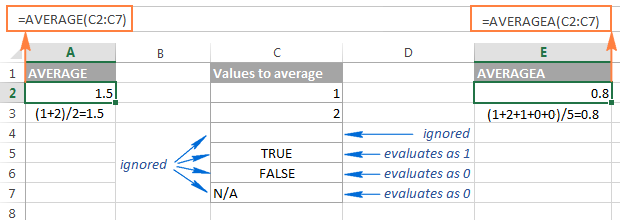So, if you do not want to include the Boolean values and text strings in your calculations, use the Excel AVERAGE function rather than AVERAGEA.

## Excel AVERAGEIF function

The AVERAGEIF function in Excel calculates the average (arithmetic mean) of all the cells that meet a specified criteria.

AVERAGEIF(range, criteria, [average_range])

The AVERAGEIFS function has the following arguments, the first 2 are required, the last one is optional:

• Range - the range of cells to be tested against the given criteria.
• Criteria - the condition used to determine which cells to average. The criteria can be supplied in the form of a number, logical expression, text value, or cell reference, e.g. 5, ">5", "cat", or A2.
• Average_range - the cells you actually want to average (optional). If omitted, the formula will calculate an average of the values in the range argument.

The AVERAGEIF function is available in Excel 2016, Excel 2013, Excel 2011 for Mac, Excel 2010 and 2007.

### Using the AVERAGEIF function in Excel - formula examples

And now, let's see how you can use the Excel AVERAGEIF function on real-life worksheets to find an average of cells that meet your criteria.

#### Example 1. Average cells that match criteria exactly

The classical use of the AVERAGEIF function in Excel is finding an average of cells that exactly match a given criterion. In this example, let's average only the sales (B2:B8) for the Banana orders (A2:A8):

`=AVERAGEIF(A2:A8, "banana", B2:B8)`

Instead of entering the condition directly in a formula, you can type it in a separate cell and refer to that cell in your formula:

`=AVERAGEIF(A2:A8, E1, B2:B8)`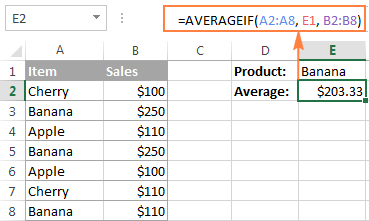Tip. To round the returned value to a certain number of decimal places, either use one of the Excel round functions to round off the actual value stored in the cell, or the Format Cells dialog to change only the display formatting.

For example, to round the average returned by the above formula to 2 decimal places, you can wrap it in the ROUND function like this:

`=ROUND(AVERAGEIF(A2:A8, "banana", B2:B8), 2)`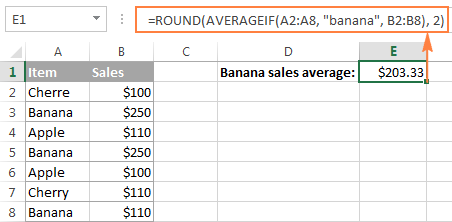Alternatively, you can select the cell with the formula (E1 in this example), press Ctrl + 1 to open the Format Cells dialog, switch to either the Number or Currency tab and select the number of decimal places you want to display. Please remember, in this case the actual value stored in a cell won't be changed, and the exact non-rounded value will be used in all calculations if you refer to that cell in other formulas.

#### Example 2. Average cells that match criteria partially (wildcard characters)

In your Excel AVERAGEIF formulas, you can use the wildcard characters in the criteria argument to average cells based on a partial match:

• Use a question mark (?) to match any single character.
• Use an asterisk (*) to match any sequence of characters.
• To find an actual question mark or asterisk, type a tilde (~) before the character in the criteria.

In the previous example, suppose you have 3 different sorts of banana and you want to find their average. The following formula will work a treat:

`=AVERAGEIF(A2:A8, "*banana", B2:B8)`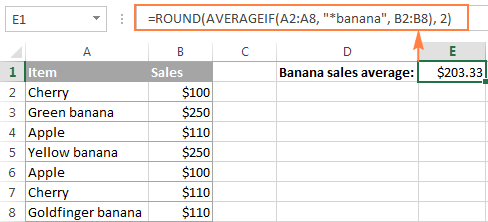If your keyword is likely to be preceded and followed by other characters, add an asterisk both in front of the word and after it, like this:

`=AVERAGEIF(A2:A8, "*banana*", B2:B8)`

To find the average of all items excluding any Banana, use the following formula:

`=AVERAGEIF(A2:A8, "<>*banana*", B2:B8)`

#### Example 3. Average cells based on numeric criteria and logical operators

Quite often, you may want to average cells where the quantity is greater than or less than a certain value. For example, we have a list of numbers in column A and we want to find an average of those that are greater than 10.

The correct way to enter such a criteria is to enclose the logical operator and the number in double quote. So, your formula for average in Excel would be as follows:

`=AVERAGEIF(A2:A7, ">10")`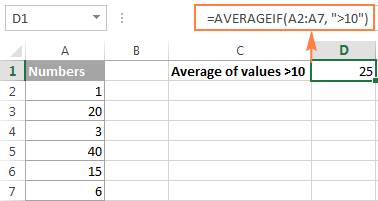Another common task is averaging numbers that are not equal to zero. For this, you would need the "not equal to" operator in the criteria argument of your AVERAGEIF formula:

`=AVERAGEIF(A2:A7, "<>0")`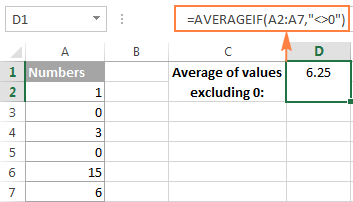As you may have noticed, we do not use the third argument [average_range] in either of the above formulas since we want to find an average in the initial range.

#### Example 4. AVERAGEIF for blank or non-blank cells

When performing data analysis in Excel, you may often need to find an average of numbers that correspond either to empty or non-empty cells.

##### Average if blank

To include blank cells that contain absolutely nothing (no formula, no zero length string), enter "=" in the criteria argument.

For example, the following formula calculates an average of cells C2:C8 if a cell in column B in the same row is absolutely empty:

`=AVERAGEIF(B2:B8, "=", C2:C8)`

To average values corresponding to visually blank cells including those that contain empty strings returned by other functions (for example, cells with a formula like =""), use "" in criteria. For example:

`=AVERAGEIF(B2:B8, "", C2:C8)`

##### Average if not blank

To find an average of values corresponding to non-empty cells, type "<>" in criteria.

For instance, the following AVERAGEIF formula calculates an average of cells C2:C8 if a cell in column B in the same row is not blank:

`=AVERAGEIF(B2:B8, "<>", C2:C8)`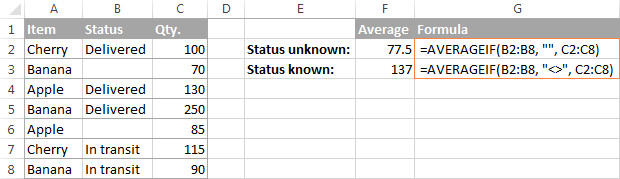#### Example 5. Using cell references and other functions in AVERAGEIF's criteria

Instead of typing the criteria in a formula, you can refer to a certain cell where your users can input different values without altering your AVERAGEIF formula.

In case a cell reference is an exact match criteria, simply type it in the criteria argument like we did in Example 1:

`=AVERAGEIF(A2:A8, E1, B2:B8)`

If you use a logical expression with a cell reference or another function in criteria, then you have to enclose the logical operator in "double quotes" and add ampersand (&) to concatenate a cell reference or function.

For example, to calculate average sales (C2:C8) that are greater than the value in E4, use the following formula:

`=AVERAGEIF(C2:C8, ">"&E4)`

With dates in B2:B8, the below formula returns the average of sales (C2:C8) that we made up to the current date:

`=AVERAGEIF(B2:B8, "<="&TODAY(), C2:C8)`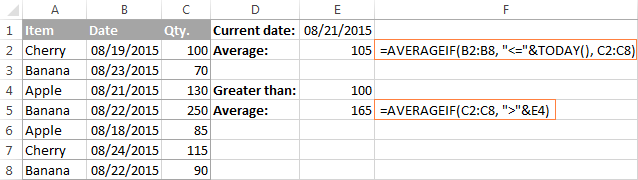## Excel AVERAGEIFS function

The AVERAGEIFS function in Excel is a plural counterpart of AVERAGEIF. It allows for multiple conditions and returns the average (arithmetic mean) of cells that meet all of the specified criteria.

AVERAGEIFS(average_range, criteria_range1, criteria1, [criteria_range2, criteria2], …)

The AVERAGEIFS function has the following arguments:

• Average_range - the range of cells that you want to average.
• Criteria_range1, criteria_range2, … - 1 to 127 ranges to be tested against the specified criteria. Criteria_range1 is required, subsequent ones are optional.
• Criteria1, criteria2, … - 1 to 127 criteria that determine which cells to average. The criteria can be supplied in the form of a number, logical expression, text value, or cell reference. Criteria1 is required, additional criteria are optional.

The AVERAGEIFS function is available in Excel 2016, Excel 2013, Excel 2011 for Mac, Excel 2010 and 2007.

### Excel AVERAGEIFS formula examples

As already mentioned, the Excel AVERAGEIFS function finds average of cells that meet all of the criteria that you specify (AND logic). In essence, you use it similarly to AVERAGEIF, except that you can supply more than one criteria_range and criteria in a formula.

#### Example 1. Average cells by multiple criteria (text and number)

Supposing that you have a list of items in column A and sales amounts in column B, let's find out an average of Banana sales that are greater than \$100.

In this AVERAGEIFS formula:

• Average_range is B2:B8 (cells that you want to average if both conditions are met);
• Criteria_range1 is A2:A8 (Items column) and criteria1 is "banana";
• Criteria_range2 is B2:B8 (Sales column) and criteria2 is ">100".

By assembling the above components together, we get the following formula:

`=AVERAGEIFS(B2:B8, A2:A8, "banana", B2:B8, ">100")`

And if you replace the actual values with cell references in your formula, you will get something similar to this: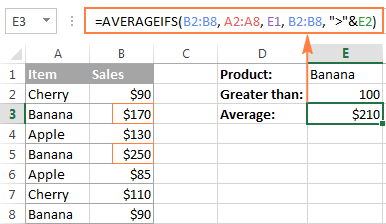As you see, only two cells (B3 and B5) meet both conditions, and therefore only these cells are averaged.

#### Example 2. Average cells based on date criteria

In this example, let's find an average of items delivered prior to 21-Aug-2015 whose status is defined (a cell in the corresponding column is not empty). With quantity listed in column D (average_range), dates in column B (criteria_range1) and status in column C (criteria_range2), the formula is as follows:

`=AVERAGEIFS(D2:D8, B2:B8, "<8/21/2015", C2:C8, "<>")`

In criteria1, you enter a date preceded with a comparison operator. In criteria 2, you type "<>" that tells the formula to include only non-empty cells within citeria_range2 (column C in this example).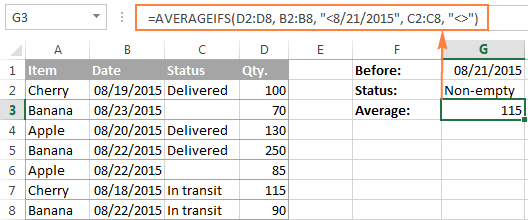Tip. When you use a number or date in conjunction with a logical operator in AVERAGEIFS' criteria, you enclose this combination in double quotes like "<8/21/2015".

### AVERAGEIF and AVERAGEIFS functions - things to remember!

Excel AVERAGEIF and AVERAGEIFS functions have much in common, in particular:

• In the average_range argument, empty cells, Boolean values of TRUE/FALSE and text values are ignored.
• In the criteria / criteria_range argument, empty cells are treated as zero values (0).
• If average_range contains only blank cells or text values, both functions return the #DIV0! error.
• If not a single cell meets the criteria (all of the criteria in case of AVERAGEIFS), the #DIV0! error is also returned.

AVERAGEIF specificities

• Average_range does not necessarily have to be of the same size as range. However, the actual cells to be averaged are determined by the size of the range argument. In other words, the upper left cell in average_range is treated as the beginning cell, and includes as many columns and rows as contained in the range argument.

AVERAGEIFS specificities

• Unlike the AVERAGEIF function, AVERAGEIFS requires each criteria_range to be of the same size as average_range.

## How to average cells by multiple criteria with OR logic

Since the Excel AVERAGEIFS function works with the AND logic and the AVERAGEIF function allows for 1 criterion only, we will have to make up our own formula to average with the OR logic. In other words, we will make a formula to calculate average in Excel if any of the specified conditions is met.

#### Example 1. Average with OR logic based on multiple text criteria

Supposing you want to get a sales average (C2:C8) both for banana and apple (A2:A8). To calculate this, you would need an array formula comprising a few Excel functions:

`=AVERAGE(IF(ISNUMBER(MATCH(A2:A8, {"banana", "apple"},0)), B2:B8))`

For convenience, you can of course replace the hardcoded criteria with corresponding references:

`=AVERAGE(IF(ISNUMBER(MATCH(A2:A8, E1:E2, 0)), B2:B8))`

Please remember that array formulas need to be entered via Ctrl + Shift + Enter, not just ENTER.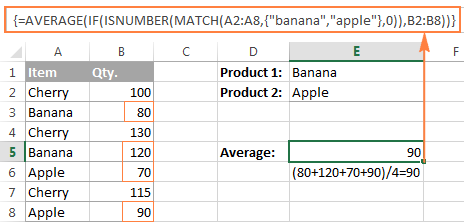Of all Excel average formulas discussed so far, this is the trickiest one (though, there are two more examples left ;) The following pattern will make it easier to adjust the formula for your own worksheets:

=AVERAGE(IF(ISNUMBER(MATCH(range, {"criteria1", "criteria2",…}, 0)), average_range))

How this formula works:

For our curious and thoughtful readers who want not only to use a formula but understand what they are doing, here's a detailed explanation of the formula's logic.

At the core of this formula, the IF function determines which values in the source range match any of the specified criteria and passes those values to the AVERAGE function. Here's how:

The MATCH function uses the source data in A2:A8 as the lookup values and compares each of those values against the lookup array represented by the criteria in E1:E2. The 3rd argument (match_type) set to 0 instructs the formula to look for exact matches:

MATCH(A2:A8, E1:E2, 0)

When a match is found, the formula returns its relative position in the lookup array, otherwise an #N/A error.

{#N/A;1;#N/A;1;2;#N/A;2}

The ISNUMBER function converts any numbers to TRUE and errors to FALSE:

{FALSE;TRUE;FALSE;TRUE;TRUE;FALSE;TRUE}

This array goes to the logical test of the IF function. In the full form, the logical test should be written like this:

IF(ISNUMBER(MATCH(A2:A8, E1:E2, 0))=TRUE

For the sake of brevity, we omit the =TRUE part because it's implied. Anyway, as the value_if_true argument of IF is set to B2:B8 and value_if_false is omitted, the IF function replaces the positions corresponding to TRUE in the array returned by ISNUMBER (please see above) by the actual values from B2:B8:

{FALSE;80;FALSE;120;70;FALSE;90}

This final array is passed to the AVERAGE function that calculates an average of numbers ignoring logical values.

#### Example 2. Average with OR logic based on numeric criteria with comparison operators

If you want to average cells based on several numeric criteria and greater than / less than conditions combined with OR logic, the formula discussed in the previous example won't work because you cannot fit those logical expressions into an array. The solution is using the SUM function in an array formula.

Supposing you have Qty. in column B and Sales in column C, and would like to average Sales that have a value greater than 50 either in column B or C. At that, you want to avoid duplicates, i.e. don't count a row twice because it has value greater than 50 both in column B and D.

Here's the formula that works a treat:

`=SUM(IF(--((C2:C8>50)+(D2:D8>50))>0,D2:D8,0)) / SUM(--(((C2:C8>50)+(D2:D8>50))>0))`

Remember, it's an array formula, and therefore you should press Ctrl + Shift + Enter to enter it correctly.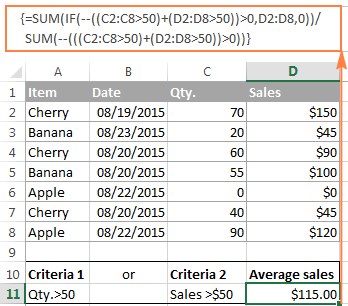As you can see, the formula consists of 2 parts. In the first part, you use the IF function with the OR statement in the logical_test argument (C2:C8>50)+(D2:D8>50). As you probably know, in array formulas, plus (+) acts like an OR operator (for more details, please see AND and OR operators in Excel array formulas). So, the first part of the formula adds up the values in column C if either condition is met. The second part returns the count of such cells, and then you divide the sum by the count to find the average.

And naturally, you can specify a different condition for each range. For example, to average sales if column C is greater than 50 or column D is greater than 100, use the following expressions: (C2:C8>50)+(D2:D8>100). The entire formula would look as follows:

`=SUM(IF(--((C2:C8>50)+(D2:D8>100))>0,D2:D8,0)) / SUM(--(((C2:C8>50)+(D2:D8>100))>0))`

#### Example 3. Average with OR logic based on blank / non-blank cells

The average formula with multiple OR criteria corresponding to blank and non-blank cells is very similar to the one we have just discussed.

##### Formula for non-blank cells

The following array formula finds the Qty. average (column B) if either a date (col. B) or status (col. C) is listed, i.e. if column B or C is not empty.

`=SUM(IF(--((B2:B8<>"") + (C2:C8<>""))>0,D2:D8,0)) / SUM(--(((B2:B8<>"") + (C2:C8<>""))>0))`

To make the formula more compact, you can concatenate the ranges using an ampersand (&):

`=SUM(IF((B2:B8&C2:C8)<>"",D2:D8,0)) / SUM( --((B2:B8&C2:C8)<>""))`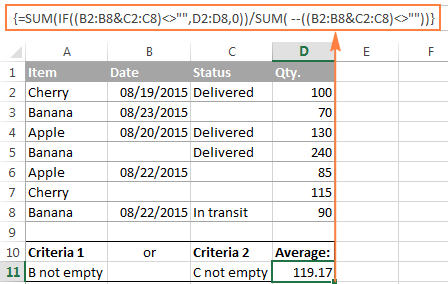##### Formula for blank cells

To average values in column D corresponding to empty cells either in B or C, replace the non-blank operator (<>"") with blank operator (=""). Concatenating the ranges with an ampersand won't work in this case. This is also an array formula, so remember to press Ctrl + Shift + Enter, not just Enter.

`=SUM(IF(--((B2:B8="") + (C2:C8=""))>0,D2:D8,0)) / SUM(--(((B2:B8="") + (C2:C8=""))>0))`

This is how you calculate average in Excel. In the next article, we will discuss a couple of formulas find weighted average, and you might be surprised to know that it's much easier than it sounds. I thank you for reading and hope to see you on our blog next week!

Excel Average formula examples

## You may also be interested in

### 81 responses to "How to calculate average (mean) in Excel - AVERAGE, AVERAGEIF, AVERAGEIFS formula examples"

1.k says:

typogrphical error under Excel Average function, second sentence, with bold-face type font

2.k says:

range is spelt as rage

•Svetlana Cheusheva (Ablebits.com Team) says:

Thank you! Fixed.

•grammarnazi says:

**spelled

3.Pieter says:

Dear Svetlana,

I need to average on a averageifs formula for a range that includes na() and Div/0!

=AVERAGEIFS('SQL Data'!\$L\$4:\$L\$65,'SQL Data'!\$A\$4:\$A\$65,'sql vs rbct'!\$B19,'SQL Data'!\$B\$4:\$B\$65,""&'sql vs rbct'!\$C19)

This formula above averages between dates and times for the selected date and time range. The data to average is in column l, data in A, times in B.

I have tried the change below but get a value error if I combine the two formulas...

{=AVERAGE(IF(ISNUMBER(Q188:Q194),Q188:Q194))}

Can you help?

•Maria Azbel (Ablebits.com Team) says:

Dear Pieter,

For us to be able to assist you better, please send us a small sample table with your data in Excel to support@ablebits.com. Please also include the expected result. Thank you.

4.Thom says:

Svetlana, I have a large data set of over 500 referential urls. The table contains both a count of page views and times spent on the page. I have the following formula that allows me to create a report that gauges interest by keyword in the urls. I need a formula which will filter the following conditions I used in my sumproduct formulat but which will give me an average instead of a sumproduct. Everything I have tried to make this happen has not worked. How can I use what you write above to accomplish this task. Your help would be appreciated. Many Thanks.

=SUMPRODUCT(('RAW DATA'!\$A\$2:\$A\$25000=B\$336)*
('RAW DATA'!\$E\$2:\$E\$25000="micrositeABC")*
(MMULT(0+ISNUMBER(SEARCH(
{"rebate","savings"},
'RAW DATA'!\$J\$2:\$J\$25000)
),{1;1})>0),'RAW DATA - PAGE CATEGORIES'!\$K\$2:\$K\$25000)

•Maria Azbel (Ablebits.com Team) says:

Hello, Thom,

For us to be able to help you better, we need the original math formula you use. Or your workbook with the expected result. You can email the details at support@ablebits.com.

•Rosie says:

– thanks for dropping by man! I like that you bring in a number of other areas and tie it into creation, I completely agree that this is the mode and task of the church today — to create a forum and median for pattpciiarion [in the Kingdom of God].

5.Alejandro says:

Hello,

I need to find the average but I need the average of the values between two other values, this lasts change with every new range

Thanks,

6.Kofi Lartey says:

I am working with data spread over 30years and need to find the average for figures which are above 0.5 in the data. I have tried using the averageifs but keeps receiving error message. Attached is a screen shot. Kindly advice how i can proceed.

YEAR MONTH DAY 1 DAY 2 DAY 3 DAY 4 DAY 5 DAY 6
1972 01 0,0 0,0 0,0 0,0 0,0 0,0
1972 02 0,0 0,0 0,0 0,0 0,0 0,0
1972 03 0,0 0,0 0,0 0,0 2,5 0,0
1972 04 0,0 25,2 0,0 0,0 0,0 59,4
1972 05 0,0 0,0 34,0 1,0 0,0 0,0
1972 06 0,0 0,0 0,0 0,0 0,0 0,0
1972 07 0,0 0,0 0,0 0,0 0,0 0,0
1972 08 0,0 0,0 0,3 0,5 0,3 0,0
1972 09 0,0 0,0 0,0 20,3 0,0 0,3

7.DIVAKAR says:

A 55
B 60
A
B 20
A
B 30
A 20
AVERAGE

8.Jackie says:

Good morning,

We are trying to use the averageifs function over an array of number from C2:O1090 - is this possible as all examples point to a column average only?

Thank you.

•Svetlana Cheusheva (Ablebits.com Team) says:

Hello Jackie,

Thank you for your question! Until now I haven't realized that all the examples in this tutorial are for a column average, maybe because it's the most typical task.

In fact, you can supply absolutely any range to your Average formula, and even several ranges or individual cells separated by commas. So, the following formula will work just fine:
=AVERAGE(C2:O1090)

•Siva says:

Is there any way to use the range in AVERAGEIFS? I have a block of data and need to take the average based on a column criteria and row criteria. Thanks.

9.ashish Kumar says:

80 Fail 92 Pass #DIV/0! #DIV/0!

How get the average and sum this range ?

10.James Arnold says:

Hi

How can I make the averageifs between two dates work if the average range and the criteria range are in different tabs to the date references?

=AVERAGEIFS('Timeliness SP'!\$D\$2:\$D\$10000,'Timeliness SP'!\$AM\$2:\$AM\$10000,">="&B66,'Timeliness SP'!\$AM\$2:\$AM\$10000,"<"&B67)

This returns Div/0 but if I look at only one date (there are multiple items per date) then it works fine.

Thanks for any help!

11.Jorge Ramos says:

I'm try to create a formula that takes a percentage in four cells per page up to 6 pages. So with a possibility of 24 total entries. that I fill in weekly. Lets say I only have 18 entries that week. So I will like the formula to see that there is only 18 entries and give me the average percentage on those 18 entries not the total 24, the rest will be zero. In the current page the cells are position in (H18,B18,B5,H5) location.

12.Anvin Antony says:

Hi I want include the Zeros between Feb to Aug and exclude the other Zeros for Calculating the Average
Jan Feb Mar Apr May Jun Average
0 505 0 0 346 0 ??

13.Daniel says:

Hi,

Within Cells A33:A151, I have options of saying either "LISTING" or "DBL END".

In cell N152, my current formula is as follows:
=AVERAGEIF(A33:A151,"LISTING",N33:N151)

This formula works, however, I am having trouble writing the formula so that when a cell within A33:A151 says "DBL END" (as opposed to "LISTING"), Cell N152 still calculates accordingly.

Thanks.

14.Nor bahadur subba says:

I really like excel, I always try to something new and to learn and this for svetlana Cheusheva...

15.Steven says:

I still dont have the answer which l am looking for:

I need a function as if 5 Days average of a certain is 59% and the sixth day l add 30% manually so then its 50% how to bring this in a function

16.Carrie says:

=AVERAGEIF(Q2:Q3304, Q2, H2:H3304)
The above formula works for the first of 3304 rows of data. I need to copy the formula down to average the remainder of the scores on each row. I need the middle indicator "Q2" to change based on the row number, but I need the other values to remain the same Q2:Q3304 and H2:H3304.

When I copy the rows down, it wants to change the beginning row for each line.
Ex:
Coping down incorrectly changes it to: =AVERAGEIF(Q3:Q3305, Q3, H3:H3305)--
I need it to be: =AVERAGEIF (Q2:Q3304, Q3, H2:H3304)

Is there a way to do this? Please assist.
Thank you!

17.Paulino Puente says:

I have this formula:

=IFERROR(AVERAGEIFS(Data!G:G,Data!B:B,">=7/1/2016",Data!B:B,"<=7/31/2016",Data!E:E,'Pareto Osmosis'!A33),"-")

I need that this formula change the values of the month that i have in the archive (with respect to column), and, i want to do a little macro to update to the next month. What do i need to do?

18.Gaurav Kumar Sharma says:

Start Down time End Down Time
11:00 AM 11:05 AM
1:00 PM 1:10 PM
2:30 PM 2:45 PM

Q Write a function in highlighted green cell to get average down time.

19.Donna says:

I have the following formula which works perfectly, however if the lookup value returns no data, I want it to average cells in the previous five rows. Can you help me with a formula to make it work? I have excel 2010

=+IFERROR(VLOOKUP(\$A6,\$A\$4:\$N\$34,9,0),0)

20.Peter O says:

For relative newcomers or those returning after a long period of non use of Excel, it might have been better to keep it really simple.
I thought it would be straight forward but alas despie reading several internet "help" sources I am no nearer to doing something very simple.
I just have about 650 temperature readings in a column of several thousand.
The range of the 650 is contiguous. I wast the arithmetic average.
"Average(C68:C720)" was the formula I attempted but nothing happens when I press enter.
If it's easy why does this not work, & why if there is "a secret" is it not mentioned?

21.Karon says:

Helo every one.
I'm trying to ge the average for a type of subscription that has 2 names (both are in the same column):
Sub1 and Sub2
(I would like the average for both types together)
However, I also want to restrict to the length of time the subscription has been held ie between two values, say 1 - 3 months.
I think what I'm after is getting the average for OR, and then an AND

Regards

Karon

22.Avnish says:

Hi,
I want to do average of the values using averageif formulae, these values contain 0 also but I want to exclude these Os from the formula.
Please suggest which formula to use.

•Svetlana Cheusheva (Ablebits.com Team) says:

Hello Avnish,

Use the AVERAGEIFS function and include "is not equal to 0" ("<>"&0) as one of the criteria.

23.Well here is a real one. says:

I need to take the average of a large data set, for example for the hour(C) 7 of day(B) 2, hour 8 of day 2 and so on. As you can see some hours have more data points than others. I have to do this for both value rows D and E.

=AVERAGEIF(\$C\$1:\$C\$33,7,D2:D33)
But then I have to manually change the range since it repeats (changes day at ROW34).
I Figured that by making a new column where the hour (C) exists regardless of whether or not it is in the data then I can skip on step:

Make column K - K1 to K24, then in any empty column:
=AVERAGEIF(\$C\$1:\$C\$33,\$K1,D\$2:D\$33)

Then by going down with the expansion cross I will get all the D averages for exactly one day, going sideways will give me the E averages as well, where no data exists I get 0#DVID! which is okay, Still I have to change the ranges and repeat once the day moves on, this works for the mean time as I only have to do one month for now, but eventually I need to do all 365 days.

If you could let me know how to include the B column so this is quicker I would appreciate it very much.

I have a vast range, I am copying two days out of 365:

A= Cell number
B= Day
C= Hour
D&E to average based on B and C:

A B C D E
01 2 07 1.01 116.01
02 2 07 0.66 119.42
03 2 08 0.62 119.49
04 2 08 0.62 120.19
05 2 08 0.57 146.63
06 2 10 0.62 18.22
07 2 11 0.66 33.57
08 2 11 0.74 28.69
09 2 11 0.88 186.97
10 2 11 1.01 131.53
11 2 11 1.05 72.37
12 2 12 1.4 153.87
13 2 12 1.01 121.94
14 2 12 1.27 156.32
15 2 12 1.62 169.6
16 2 13 1.67 161.02
17 2 13 0.83 185.81
18 2 14 1.01 154.28
19 2 15 2.72 166.47
20 2 15 1.36 159.9
21 2 15 2.1 155.9
22 2 15 1.97 166.12
23 2 16 1.01 165.87
24 2 16 2.14 146.66
25 2 16 1.48 147.16
26 2 16 1.27 143.99
27 2 17 0.78 201.54
28 2 17 0.88 9.34
29 2 17 1.23 165.03
30 2 17 1.53 160.04
31 2 17 0.88 147.54
32 2 17 1.01 13.76
33 2 18 1.09 26.9
34 3 07 1.32 147.47
35 3 07 0.92 147.65
36 3 08 0.53 147.75
37 3 08 0.62 147.65
38 3 08 0.57 147.54
39 3 10 2.14 96.31
40 3 10 2.14 89.78
41 3 10 2.8 84.44
42 3 10 2.88 81.74
43 3 10 1.93 97.05
44 3 11 1.83 92.31
45 3 11 2.37 88.98
46 3 11 2.45 87.96
47 3 11 1.93 87.89
48 3 11 2.67 86.13
49 3 11 2.32 89.54
50 3 12 2.02 91.15
51 3 12 5.12 84.48
52 3 13 4.51 76.86
53 3 13 5.12 73.91
54 3 13 4.68 79.71
55 3 13 4.24 79.71
56 3 13 3.23 78.72
57 3 14 1.48 58.32
58 3 14 1.88 60.64
59 3 15 1.44 24.3
60 3 15 2.28 55.4
61 3 15 3.46 61.02
62 3 15 4.77 67.24
63 3 15 2.98 65.73
64 3 16 2.41 72.05
65 3 16 2.23 89.26
66 3 16 2.76 76.4
67 3 16 4.28 81.39
68 3 16 3.72 76.09
69 3 16 3.46 72.37
70 3 17 3.07 76.86
71 3 17 4.24 95.85
72 3 17 3.58 99.26
73 3 17 3.89 85.08
74 3 17 2.88 90.76

This was the easy one... now I have to add a column for the minutes, where again the some minutes are missing... so help would be appreciated.

24.Md. Mahbubur Rahman says:

Hi, Could you please tell me how can i calculate average excluding blank boxes in excel?

•Svetlana Cheusheva (Ablebits.com Team) says:

Hi!

By default, the Excel Average function excludes blank cells from an average, though cells with zero values are included.

25.Jonny says:

I am using an AVERAGEIF formula using ranges that have been rounded up and down. When I evaluate my formula the range changes from say 9.0 to 8.999999999 which then means the formula produces a #DIV/0! value.

Can you help with sorting this out please?

•Jonny says:

Sorry, that should have read AVERAGEIFS

Cheers

26.ultiabaz36 says:

I need formula to calculate this data
67 87 86 57 97 86 54 87
determine if the candidate pass PR not if the average score is 55

27.PR says:

I want to average multiple cells in a row for each task and then copy down the formula rows below. Each cell has a text value which first needs to be converted to number and then the average calculated. E.g. A1="Start" (which equals 0; B1="Mid Point" (which equals 50) and C1="End" and then Column D1=Average(A1:C1) = ((0+50+100)/3)=50. Formula should work for a range of cells without including the condition (if cell="Start",0,if(cell="Mid Point",50,100))for A1,B1...M1 separately.

28.khalid says:

2001 8.5
2002 9
2003 10
2004 18
2005 16
2006 10
2007 11
2008 16.25
2009 14.15
2010 15.5
2011 25
2012 15.5
2013 15.35
2014 13.65
2015 11.86
2016 5.15

i want to calculate the average among the years like 2003-2009 or 2011-2015 etc.
from: 2003 is one cell
to: 2009 is on cell

i m using =AVERAGEIFS(B7:B22,A7:A22,B30&"=>"&"="&B31)
BUT NOT ANSWERING, PLEAE HELP ME IN THIS MATTER.

29.Cool says:

Hi Team Ablebits,

can i use AverageIf function for the criteria by font color?

30.LD says:

I have the monthly production but I want to average the documents processed by user per hour
I have following information:
User name Posting Date Time Document no.
1 1/17/2017 13:53:27 xxxxx
1 1/18/2017 9:24:29 xxxxx
2 1/17/2017 15:05:30 xxxxx
3 1/20/2017 12:09:51 xxxxx
4 1/01/2017 12:09:52 xxxxx
5 1/31/2017 08:30:20 xxxxx

hour
have a file with the monthly production and I´m trying to average the invoices process by user per day

31.Jide says:

I want to auto calculate average of every five cells of a column to another column. I have daily rainfall data for 30 years but I want average of every 5 days of each year.

32.MITCH says:

hi, i need to get the average of below data but it seems like im having problem, just one category from type is getting. THANKS SO MUCH IN ADVANCE:)

here's my formula: =AVERAGEIFS(D:D,B:B,"prior20",C:C,"cavite",E:E,{"crtfixed","crtvoice","rbg-crt"})

A B C D
date area ave type
prior20 cavite 10 crtfixed
prior20 cebu 9 crtvoice
prior20 makati 8 rbg-crt
prior20 cavite 7 crtfixed
prior20 cebu 6 crtfixed
prior20 makati 5 crtvoice
prior20 cavite 4 rbg-crt
prior20 cebu 3 crtfixed
beyond luzon 2 crtfixed
prior20 makati 5 crtvoice
prior20 cavite 4 rbg-crt
prior20 cebu 3 crtfixed

•Natalia Sharashova (Ablebits.com Team) says:

Hi Mitch,
try this formula:

=SUM(SUMIFS(D:D,B:B,"prior20",C:C,"cavite",E:E,{"crtfixed","crtvoice","rbg-crt"}))/SUM(COUNTIFS(B:B,"prior20",C:C,"cavite",E:E,{"crtfixed","crtvoice","rbg-crt"}))

33.Jeff says:

Hi , i am trying to find an average but only based on a certain number of cells in a range. I want to leave the full range in place for the Average formula , but only use part of it based on criteria :

Example : Range A1:M1 carries a forecast of values at start of a Quarter. Three weeks into the Quarter , I then update with Actuals into Cells A1 , B1 , C3 (i.e. i have input 3 cells with Actuals.)

Now i want the average formula for A1:R1 to only use the first 3 cells (which are the Actuals now and the remainder are the Forecast) in my result.

34.xyz says:

Hi , i am trying to find an average based on criteria :

Example 1 : A1 = 3, E1=2 , J1 = 10, My answer will be A1+E1+J1/3 = 5.

Example 2 A1 = 3, E1 = 3, J1 = -, My answer will be A1+E1+J1/3 = 3.

Example 3 A1 = -, E1 = -, J1 = -, My answer should be A1+E1+J1/3 = 0.

Example 1 and 2 is Ok, Where as in example 3 am getting error #DIV/0! instead of this i need 0 in the box.

•Natalia Sharashova (Ablebits.com Team) says:

Hi,
please note that empty cells are ignored by AVERAGE function.
Try entering zero (0) in the cells, if you want zero to return.

Hope it helps!

35.Antje says:

Dear Svetlana & Community,
thanks for the great tutorials.
I am trying to average a selection of non-continous columns, all titled "kcal in".
I have set the columns DF2:XA2 as the range, which is the title column. "kcal in" is the crietria and DF4:\$XA\$435 is the average of all kcal in in the DF4 row. I can drag the formula down to obtain the average for rows DF5 etc.

=AVERAGEIF(\$DF\$2:\$XA\$2, "kcal in", DF4:\$XA\$435)

This works well, however, I am trying to exclude any 0 values in row DF4 from the average and I am not sure where to put this criterion.

•Antje says:

Found the answer here myself :)
=AVERAGEIFS(DF6:XA6,\$DF\$2:\$XA\$2,"kcal in",DF6:XA6,">0")

Average_range is DF6:XA6 (the row you want to average if both conditions are met);
Criteria_range1 is \$DF\$2:\$XA\$2 (the head column, range fixed with dollar sign) and criteria1 is "kcal in"(allowing to average non-continuous columns)
Criteria_range2 is DF6:XA6 (the row you want to average) and criteria2 is ">0". (values of 0 are excluded)

Thanks for the great site, finally my mind can rest :D

36.Abhijith says:

could you help me to calculate the mean with specific interval in excel.
I have daily data of rainfall for 30 years from that how could i calculate weekly mean easily

37.Vinay says:

=AVERAGE(IF(\$C\$5:\$C\$310="ACC",\$E\$5:\$E\$310,""""))

In this if there is zero value & either cell is blank in E5 to E310 then value should not be count.
"c" "D" "E" "F"
ACC 3200 120 190
ACC 3201
ACC 3400 180 200
ACC 3200
ACC 2989 140 220
ACC 3000
ACC 3050 135 210

Avg of (190,200,220 & 210) showing 82.1

Pls suggest

38.Jane says:

Would you please help me calculate the average of the number of households who treat water per each health volunteer for a duration of 3 months?

39.June says:

Can someone help me figure out what is wrong with this function:

{=AVERAGE(IF(CementByRegion!B3:B42,Sheet1!J20,CementByRegion!C3:G42))}

J20="Text" that shows up in column "B". I'm trying to do an average of data in more than one column.

•Natalia Sharashova (Ablebits.com Team) says:

Hello,

I believe this will do :)
=AVERAGEIF(CementByRegion!B3:B42,"="&Sheet1!J20, CementByRegion!C3:G42)

40.Rosh says:

hi Svetlana
can you please tell me if you can do a similar formula when you need to calculate a nested 'min if' formula and need to ignore the blank cells. This is because when I have blank cells in array of data, the result I get for min if is a blank.
Would really appreciate you help on this
many thanks
Rosh

41.aas1354 says:

I have a formula which is like this

=AVERAGEIF('sheet1'!A:A,"Truck",'sheet1'!V:V)

which is take the average of all data in column V (sheet1) based on all info related to the Truck in column A.

Now, I want this formula to read the same information but by consider a range of dates in between 1st of October to 15th (I have dates in Column B in the same sheet1).

The formula I created for the second part is like this, but can not merge it to the first part:

'sheet1'!B:B,">="&P2,'sheet1'!B:B,"<="&P3

P2= Start date
P3= End date

•Gennady Terekhov (Ablebits.com Team) says:

Hello,

=AVERAGEIFS('sheet1'V:V,'sheet1'A:A,"Truck",'sheet1'B:B," > ="&P2,'sheet1'B:B," < ="&P3)

•aas1354 says:

Hello and Thanks for your feedback,
It apparently do something, but gave me #DIV/0! error, it should give me a 2.53.

Any input?

•Gennady Terekhov (Ablebits.com Team) says:

Hello,

1 The ">=" и "<=" conditions in the formula don't contain any spaces.
2 The P2 and P3 cells in your table contain dates.
3 The 'sheet1'!V:V range for calculating an average value is not empty or doesn’t contain text values.
4 The 'sheet1'!A:A and 'sheet1'!B:B ranges with selection criteria are not empty as well.

42.aas1354 says:

Hello,
All checked and good to go, but still shows #DIV/0! error instead of 2.53 which I calculated manually.
Any other input or any other way to write the formula.
Cheers,

43.aas1354 says:

Supper thanks finally works :)
an error (,) somewhere in formula :) :) :)

44.aas1354 says:

Now any input for Max (IF) formula:

=MAX(IF('sheet1'!A:A=J4,'sheet1'!T:T))

In above formula, it consider the Max in column T, by returning name "Truck" in column A. // J4=Truck

Now, if I want to add that dates frame (formula part), how should I go through:

'sheet1'!B:B,">="&P2,'sheet1'!B:B,"<="&P3

P2= Start date
P3= End date

•Gennady Terekhov (Ablebits.com Team) says:

Hello,

=MAXIFS('sheet1'!T:T, 'sheet1'!A:A, J4, 'sheet1'!B:B, ">="&P2, 'sheet1'!B:B, "<="&P3)

45.Najam bilal says:

i need Average
=AVERAGEIF('(Sheet 1)'!A1,'(Sheet 2)'!A1,">1%")
but this formula not put what i do? i need Average different sheets and i want to 0 no count in average.
Like= 0,11,0 =11 Average
in this condition 0 value no coun

46.Ramaraj says:

Dear Svetlana,

I learned some shortcuts. I would like to learn the Macro as well. I hope u can suggest better way to learn the Macro.

Kind Regards,
Ram.

•Svetlana Cheusheva (Ablebits.com Team) says:

Hi Ramaraj,

We have a couple of tutorials on Excel macros. Please check out these ones:

47.Chad says:

I need help with the following formula. Any suggestions would be appreciated. =AVERAGEIFS(E:E,"=<99.9",M:M,"*A-Shift*")

48.Roy says:

I was able to use your help to write a rolling average of my income for the past year, thanks a bunch!

49.Chris says:

I have multiple columns of dates in excel and am trying to find an average of workdays between the columns. I want Sheet1 to be my trending sheet and Sheet2 to be the data sheet. Column R: Receipt Date, Column S: Processed Date, Column T: Routed Date, Column U: Approved Date. I want the average over the entire columns. Because they are lifecycle dates, some cells may be blank. For example, something may be processed but not yet routed, another item may be processed, routed, and approved, etc.

I need the calculation to show me overall for those items that are both (for example) received and processed, how many days on average it takes to complete processing once the item has been received, etc. (excluding blanks e.g. those items that have neither been received or processed and received but not yet processed)

Right now my calculation is just looking at Column S17:T24 (for troubleshooting and testing) on Sheet 2 and it's "mostly" working and correct but not fully. Once it's fully working and correct I want to move the calculation off to the trending sheet and refer back to the data sheet.

So I have two issues:
1.) I need the calculation to be fully correct.
2.) I need it to be able to refer successfully to another worksheet in the same workbook.

My current calculation is: {=AVERAGE(IF(NETWORKDAYS(S17:S24+0,T17:T24+0)>0,NETWORKDAYS(S17:S24+0,T17:T24+0),-1))}

50.Pablo says:

Hi Oscar,
My is Pablo and I would like to ask you about this situation. I have got a column with several values and some of them are zeros. As they are dB measurements this is the array formula I use to get the average: =10*LOG(AVERAGE(10^(C3:C66/10)))

My problem is that I am trying to get with a formula that does not take in account the zeros.

I have tried the next formula but it seems that does not work for my situation: =10*LOG(AVERAGEif(C3:C66,"0",[10^(C3:C66/10)]))

It would be very apprecited if you could give me a hint to solve this problem.

Pablo.

51.abbasi says:

please sir i need a formulas basic because i learned first time but i want to learned excel formula

52.Alexa says:

Hi!

I have a daily work in regards with excel, we had an instruction that goes like this "If the sale of this person is below the average of everyone, the commission must be 5%, and 10% if above the average all.
In the column, the result must be either 5% or 10%." I really am having a hard time using the right formula.

Thank you!

53.Nicci Robinson says:

I've got an averages calculation with every other non-adjacent cell
=AVERAGE(B5,D5,F5) etc currently up to SN5 which is 255 numbers. When I try to add the next one in, I keep getting "You've entered too many arguments for this function" but there are no arguments, it's just an average. I've checked to make sure there are no cells in the calculation that shouldn't be there, and everything looks as it should be. Is there a maximum on the number of data cells in an averages calculation? Surely not? Please help!!

54.Scholar says:

I am working working on data in 2 sheets with similar dimensions.
The data is for many countries, and stretches over 22 years.
Sheet 1 categorises data into 3 codes: 0, 1, 2 (0=not traded at all; 1=traded at begining; and 2=not traded in the last 2 years)
Sheet 2 contains values values (exports) corresponding to cartegories 0, 1 and 2.

Now, I would like to find average values for each cartegory. In other words I am trying to find the average for Cartegory 0; Cartegory 1 and Cartegory 3. How do I find the average.

Thank you.

55.Vishavnath Pratap Singh says:

Hello,
I need your help, one excel in this formula use, after use this formula i am unable to use this formula.
=IF(F7<1,"87",IF(F7<2,"85",IF(F7<3,"82",IF(F7<4,"82",IF(F7<5,"78",IF(F7<6,"75",IF(F7<7,"72")))))))

56.Vijay says:

I want to perform all the calculations in one cel,item,Quantity and Moisture weighted average in exal

57.David says:

Is there a formula in MS-Excel 2016 that can provide an average if every other cell is a percentage? Only want the averages of the percentages for a specific cell range

58.Sean says:

I am trying to average between a set range. I want to exclude anything below 10 and over 70.
Example
50
8
6
40
80

The average I want to get is 45 but cant find a formula to do this. Please help.

59.MUZEKKER says:

Hi,
I am trying to have AVERAGE of a column with AVERAGEIFS option. It gives me error with below formula. It gives error after use "0" at the end of formula too.
What could be the right one.
60.Caleb says: